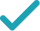Solve for c (complex solution)Solve for x (complex solution)Solve for Δ (complex solution)Solve for bSolve for cSolve for xSolve for ΔGraphGraph Both Sides in 2D
Graph in 2DStill have questions?
55b+2cx=\Delta x
Swap sides so that all variable terms are on the left hand side.
2cx=\Delta x-55b
Subtract 55b from both sides.
2xc=x\Delta -55b
The equation is in standard form.
\frac{2xc}{2x}=\frac{x\Delta -55b}{2x}
Divide both sides by 2x.
c=\frac{x\Delta -55b}{2x}
Dividing by 2x undoes the multiplication by 2x.
c=\frac{\Delta }{2}-\frac{55b}{2x}
Divide \Delta x-55b by 2x.
\Delta x-2cx=55b
Subtract 2cx from both sides.
\left(\Delta -2c\right)x=55b
Combine all terms containing x.
\frac{\left(\Delta -2c\right)x}{\Delta -2c}=\frac{55b}{\Delta -2c}
Divide both sides by \Delta -2c.
x=\frac{55b}{\Delta -2c}
Dividing by \Delta -2c undoes the multiplication by \Delta -2c.
x\Delta =2cx+55b
The equation is in standard form.
\frac{x\Delta }{x}=\frac{2cx+55b}{x}
Divide both sides by x.
\Delta =\frac{2cx+55b}{x}
Dividing by x undoes the multiplication by x.
\Delta =2c+\frac{55b}{x}
Divide 55b+2cx by x.
55b+2cx=\Delta x
Swap sides so that all variable terms are on the left hand side.
55b=\Delta x-2cx
Subtract 2cx from both sides.
55b=x\Delta -2cx
The equation is in standard form.
\frac{55b}{55}=\frac{x\left(\Delta -2c\right)}{55}
Divide both sides by 55.
b=\frac{x\left(\Delta -2c\right)}{55}
Dividing by 55 undoes the multiplication by 55.
55b+2cx=\Delta x
Swap sides so that all variable terms are on the left hand side.
2cx=\Delta x-55b
Subtract 55b from both sides.
2xc=x\Delta -55b
The equation is in standard form.
\frac{2xc}{2x}=\frac{x\Delta -55b}{2x}
Divide both sides by 2x.
c=\frac{x\Delta -55b}{2x}
Dividing by 2x undoes the multiplication by 2x.
c=\frac{\Delta }{2}-\frac{55b}{2x}
Divide \Delta x-55b by 2x.
\Delta x-2cx=55b
Subtract 2cx from both sides.
\left(\Delta -2c\right)x=55b
Combine all terms containing x.
\frac{\left(\Delta -2c\right)x}{\Delta -2c}=\frac{55b}{\Delta -2c}
Divide both sides by \Delta -2c.
x=\frac{55b}{\Delta -2c}
Dividing by \Delta -2c undoes the multiplication by \Delta -2c.
x\Delta =2cx+55b
The equation is in standard form.
\frac{x\Delta }{x}=\frac{2cx+55b}{x}
Divide both sides by x.
\Delta =\frac{2cx+55b}{x}
Dividing by x undoes the multiplication by x.
\Delta =2c+\frac{55b}{x}
Divide 55b+2cx by x.